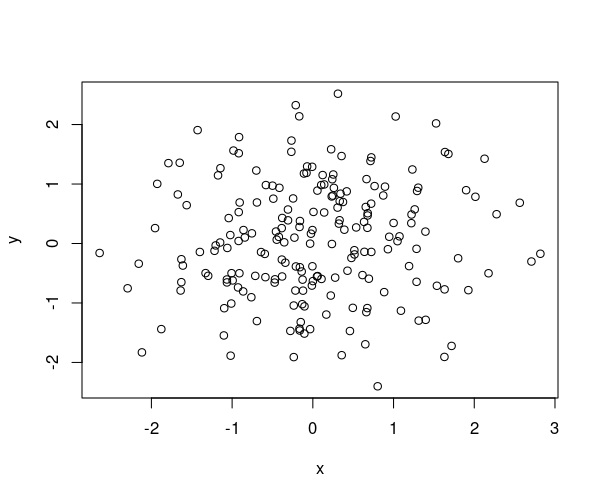## Exam 1

1. #### Question

The following figure shows a scatterplot. Which of the following statements are correct?1. The absolute value of the correlation coefficient is at least $0.8$.
2. The slope of the regression line is about $1$.
3. The standard deviation of $Y$ is at least $6$.
4. For $X=0.3$, $Y$ can be expected to be about 0 .
5. The mean of $X$ is at most $5$.

#### Solution

1. False. No association between the variables is observed in the scatterplot. This implies a correlation coefficient close to $0$.
2. False. The slope of the regression line is given by $r·{s}_{y}/{s}_{x}$ and hence not about equal to $1$.
3. False. The standard deviation of $Y$ is about equal to $1$ and is therefore smaller than $6$.
4. True. The regression line at $X=0.3$ implies a value of about $Y=0$.
5. True. The mean of $X$ is about equal to $0$ and hence is smaller than $5$.